Home    |    Teacher    |    Parents    |    Glossary    |    About UsExponents are used in many algebra problems, so it's important that you understand the rules for working with exponents. Let's go over each rule in detail, and see some examples.

Rules of 1

There are two simple "rules of 1" to remember.

First, any number raised to the power of "one" equals itself. This makes sense, because the power shows how many times the base is multiplied by itself. If it's only multiplied one time, then it's logical that it equals itself.

Secondly, one raised to any power is one. This, too, is logical, because one times one times one, as many times as you multiply it, is always equal to one.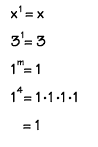Product Rule

The exponent "product rule" tells us that, when multiplying two powers that have the same base, you can add the exponents. In this example, you can see how it works. Adding the exponents is just a short cut!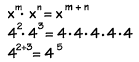Power Rule

The "power rule" tells us that to raise a power to a power, just multiply the exponents. Here you see that 52 raised to the 3rd power is equal to 56.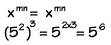Quotient Rule

The quotient rule tells us that we can divide two powers with the same base by subtracting the exponents. You can see why this works if you study the example shown.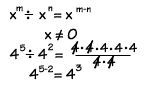Zero Rule

According to the "zero rule," any nonzero number raised to the power of zero equals 1.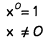Negative Exponents

The last rule in this lesson tells us that any nonzero number raised to a negative power equals its reciprocal raised to the opposite positive power.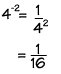Homework Help | Algebra | The Basics of AlgebraEmail this page to a friendSearch·  Properties of     Real Numbers·  Exponents·  Evaluating expressions·  Like terms·  SimplifyingFirst Glance In Depth Examples WorkoutExponents